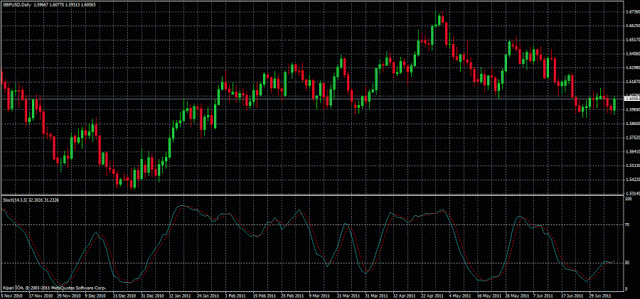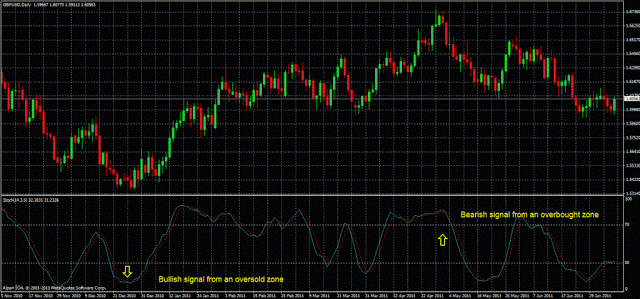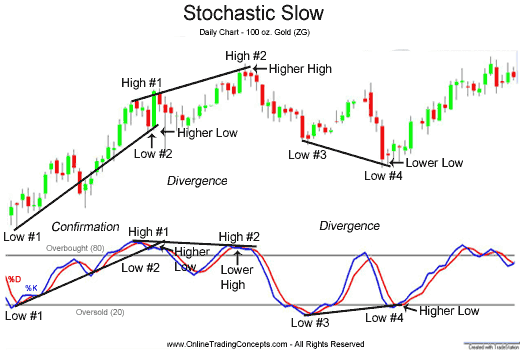# The stochastic oscillator indicator

### What is the stochastic oscillator?

A stochastic system is one that works mostly by chance. It is therefore a mathematical algorithm that deals with the processes whose evolution is random and base its results on probabilities that change over time. The fact that the probability calculations change over time is the difference with a probabilistic model that is not stochastic.

From the previous words we can deduce that the stochastic indicator will provide a value that corresponds to a probability that will help us predict the behavior of the market. The stochastic series in financial markets were applied for the first time in the 50´s (It was introduced by  George C. Lane in the year of 1950) of the twentieth century and since then the stochastic  has been  one of the most common indicators in technical analysis. According to Lane, the stochastic oscillator is not an indicator designed to follow the price, it was designed to follow the speed or the momentum of price. In general, the momentum changes direction before price.If this description is correct, this indicator should provide a valuable clue to future price direction

Stochastic is, by definition, an oscillator-type indicator which varies from 0 to 100 measuring overbought and oversold conditions in the market. The stochastic oscillator consists of two lines called %K and %D. %K line is the stochastic itself and the%D line is a moving average of% K (again we have two lines obtained from the same calculation, a slow one(% D) and fast one(% K) whose intersections can be used as signals to buy and sell).Stochastic oscillator in Metatrader 4 (MT4 platform of EasyMarkets)

The formula to calculate the %K is the following:

Where:

• H is the highest price over the last n periods.
• L is the lowest price over the last n periods.

On the other hand, the% D is usually calculated as follows: –%D = 3 period moving average of %K.

### Practical use of stochastic oscillator

The most popular form of use of the stochastic oscillator is the identification of overbought and oversold zones in the market and the crosses of the lines %D and %K. In the same way as other oscillator type indicators, it is also common to use the divergences between price and indicator.
• Crosses of %K and %D lines: As we said a few paragraphs above, the %D line is a moving average of %K line, so we can use the same concept of moving averages crossing to produce buy&sell signals to trade in the market. In the case of the stochastic indicator, the slow moving average is %D and the fast moving average is %K, so a buy signal appears when the %K line crosses from bottom to top the %D line%. Conversely, a sell signal appears when the %D line crosses from top to bottom the %K line. These signals crossing of% K and% D generate too many false signals, but rather its reliability could be increased by taking along with areas of overbought and oversold that we will see below.
• Overbought and oversold zones: When the stochastic indicator is added to a price chart is possible, among other options, choose two levels that will serve to highlight areas with overbought and oversold conditions. These levels are set by default to 80 and 20. For example, when the stochastic falls below the lower level (20), that is a clear signal that the price of a currency pair or other instrument is in oversold condition, so we can expect an upward movement in any moment. In this case, the ideal moment to open a long position is that one that coincides with the crossing of% K and% D lines from bottom to top.Trading signals of Stochastic oscillator
• Divergence of price/stochastic: Analogous to the divergence of any other oscillator (the MACD and RSI for example), sometimes the stochastic oscillator presents divergences with the price which can be bullish and bearish and can be used as signals to trade in the market.  For example, the bearish divergence, which can be taken as a signal to open a short position,  appears when consecutive highs in price are increasing and the corresponding highs in the stochastic are decreasing. On the contrary, the bullish divergence, that can be taken as a signal to open a long position,  appears when consecutive minimum in price are decreasing and the corresponding minimum in the stochastic are increasing.Divergences Price-Stochastic Oscillator